#Function Repository Resource:

# MeanDifferenceCI

Estimate the confidence interval of the difference between the population means

Contributed by: Wolfram Research
 ResourceFunction["MeanDifferenceCI"][list1,list2] gives a confidence interval for the difference between the population means estimated from list1 and list2.

## Details and Options

ResourceFunction["MeanDifferenceCI"][list1,list2] gives a confidence interval {min,max} centered at Mean[list1]-Mean[list2].
ResourceFunction["MeanDifferenceCI"] is based on a normal distribution if the population variances are assumed known.
If the variances for the two populations are assumed equal and unknown, the interval is based on a Student t distribution with Length[list1]+Length[list2]-2 degrees of freedom.
If the population variances are not assumed known and not assumed equal, Welch's approximation for the degrees of freedom is used.
The following options can be given:
 ConfidenceLevel 0.95 probability associated with a confidence interval EqualVariances False whether the unknown population variances are assumed equal KnownVariance None list of variances of each population

## Examples

### Basic Examples

The 95% confidence interval for the difference in two population means:

 In:=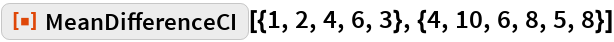Out=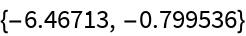### Options

#### ConfidenceLevel

The 99% confidence interval:

 In:=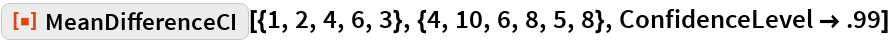Out=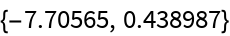#### EqualVariances

A confidence interval assuming equal but unknown variances:

 In:=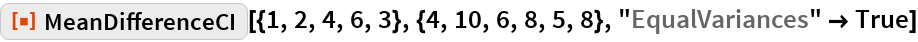Out=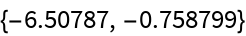#### KnownVariance

A confidence interval assuming known variances:

 In:=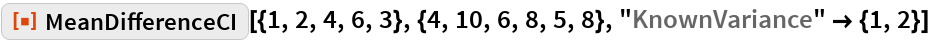Out=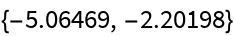## Requirements

Wolfram Language 11.3 (March 2018) or above